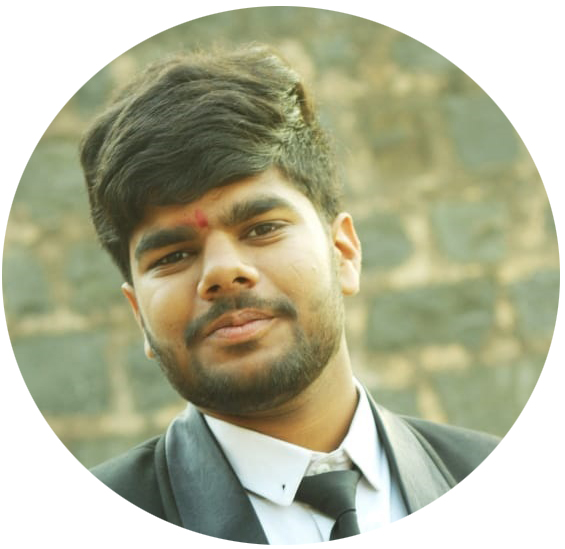Table of Content

## Introduction

In this tutorial we will be implementing human pose estimation using python as a programming language and for overlaying all the 18 skeleton points in a human body we will be using OpenCV. In addition we will use OpenCV to load all the pre-trained deep-learning architecture based on tensorflow. We have already developed a tensorflow model to train these human pose estimation, at the end of this tutorial you will be able to deploy algorithm on pre-stored images, videos and also using web-cam.

## Tools Needed

1. Install Anaconda
2. Packages of multiple Ide’s
3. Ide’s (any one)
1. Jupyter Notebook
2. Spyder
3. VSCode
4. Install these libraries
1. OpenCV
2. Pip install opencv – python

## Importing Libraries

```    import cv2 as cvv
import matplotlib.pyplot as plt

```

We need some sample still image, video and live feed to test this application. In case of still image we will be using 2 sample , one a very good input data and another a practical real life still. This would help us understand the room available for further improvement as you experiment with this. Download the images, pose estimation image sample 1 and pose estimation image sample 2.

We would also need to download pretrained weightages file. We refer to this as graph_opt.pb file in the code.

We would be using this YouTube dance video of Rhea Rai for our pose estimation on a video file. You can download by clicking dance pose track real life.

## Importing Weights of the Model

```    weights = cvv.dnn.readNetFromTensorflow("graph_opt.pb")

```

## Tuning Hyper-parameters

```    Width = 368
Height = 368
th = 0.2

PARTS = { "Nose": 0, "Neck": 1, "RightShoulder": 2, "RightElbow": 3, "RightWrist": 4,
"LeftShoulder": 5, "LeftElbow": 6, "LeftWrist": 7, "RightHip": 8, "RightKnee": 9,
"RightAnkle": 10, "LeftHip": 11, "LeftKnee": 12, "LeftAnkle": 13, "RightEye": 14,
"LeftEye": 15, "RightEar": 16, "LeftEar": 17, "Background": 18 }

PAIRS = [ ["Neck", "RightShoulder"], ["Neck", "LeftShoulder"], ["RightShoulder", "RightElbow"],
["RightElbow", "RightWrist"], ["LeftShoulder", "LeftElbow"], ["LeftElbow", "LeftWrist"],
["Neck", "RightHip"], ["RightHip", "RightKnee"], ["RightKnee", "RightAnkle"], ["Neck", "LeftHip"],
["LeftHip", "LeftKnee"], ["LeftKnee", "LeftAnkle"], ["Neck", "Nose"], ["Nose", "RightEye"],
["RightEye", "RightEar"], ["Nose", "LeftEye"], ["LeftEye", "LeftEar"] ]

```

## Importing Image

```    img = cvv.imread("pose-best-case-image-png-format.png")
plt.imshow(img)
plt.imshow(cvv.cvtColor(img, cvv.COLOR_BGR2RGB))

```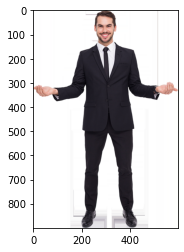Figure 1 : Pose Estimation Image Sample One

## Defining Model for Pose Estimation Using Images

```    def human_pose_estimation(image):
IWidth = image.shape
IHeight = image.shape
weights.setInput(cvv.dnn.blobFromImage(image, 1.0, (Width, Height), (127.5, 127.5, 127.5), swapRB = True, crop = False))
o = weights.forward()
o = o[:, :19, :, :]
assert(len(PARTS) == o.shape)

pnts = []
for i in range(len(PARTS)):
Map = o[0, i, :, :]
_, conf, _, point = cvv.minMaxLoc(Map)
X = (IWidth * point) / o.shape
Y = (IHeight * point) / o.shape
pnts.append((int(X), int(Y)) if conf > th else None)

for pair in PAIRS:
partF = pair
partT = pair
assert(partF in PARTS)
assert(partT in PARTS)

idF = PARTS[partF]
idT = PARTS[partT]

if pnts[idF] and pnts[idT]:
cvv.line(image, pnts[idF], pnts[idT], (0, 255, 0), 3)
cvv.ellipse(image, pnts[idF], (3, 3), 0, 0, 360, (0, 0, 255), cvv.FILLED)
cvv.ellipse(image, pnts[idT], (3, 3), 0, 0, 360, (0, 0, 255), cvv.FILLED)

t, _ = weights.getPerfProfile()
frequency = cvv.getTickFrequency() / 1000
cvv.putText(image, '%.2fms' % (t / frequency), (10, 20), cvv.FONT_HERSHEY_SIMPLEX, 0.5, (0, 0, 0))

```

## Predicting Output

```
# Pose estimation called on best case still image sample
estimated_pose = human_pose_estimation(img)
plt.imshow(cvv.cvtColor(img, cvv.COLOR_BGR2RGB))

```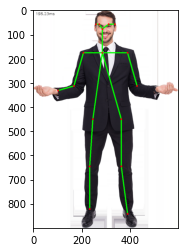Figure 2 : Pose Estimation applied on Image Sample One

```    # lets import the second sample still image file saved in jpg format
plt.imshow(img)
plt.imshow(cvv.cvtColor(img, cvv.COLOR_BGR2RGB))

```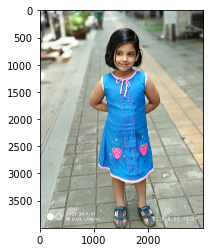Figure 3 : Pose Estimation Image Sample Two

```    # Pose estimation called on second case
estimated_pose = human_pose_estimation(img)
plt.imshow(img)
plt.imshow(cvv.cvtColor(img, cvv.COLOR_BGR2RGB))

```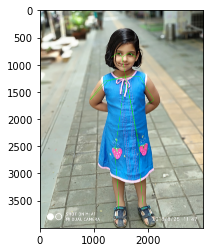Figure 4 : Pose Estimation applied on Image Sample Two

## Defining Model for Pose Estimation Using Video

```    capture = cvv.VideoCapture("dance-pose-track-real-life-mp4-format.mp4")
capture.set(3, 800)
capture.set(4, 800)

if not capture.isOpened():
capture = cvv.VideoCapture(0)
if not capture.isOpened():

while cvv.waitKey(1) < 0:
if not hasimage:
cvv.waitKey()
break

IWidth = image.shape
IHeight = image.shape
weights.setInput(cvv.dnn.blobFromImage(image, 1.0, (Width, Height), (127.5, 127.5, 127.5), swapRB = True, crop = False))
o = weights.forward()
o = o[:, :19, :, :]
assert(len(PARTS) == o.shape)

pnts = []
for i in range(len(PARTS)):
Map = o[0, i, :, :]
_, conf, _, point = cvv.minMaxLoc(Map)
X = (IWidth * point) / o.shape
Y = (IHeight * point) / o.shape
pnts.append((int(X), int(Y)) if conf > th else None)

for pair in PAIRS:
partF = pair
partT = pair
assert(partF in PARTS)
assert(partT in PARTS)

idF = PARTS[partF]
idT = PARTS[partT]

if pnts[idF] and pnts[idT]:
cvv.line(image, pnts[idF], pnts[idT], (0, 255, 0), 3)
cvv.ellipse(image, pnts[idF], (3, 3), 0, 0, 360, (0, 0, 255), cvv.FILLED)
cvv.ellipse(image, pnts[idT], (3, 3), 0, 0, 360, (0, 0, 255), cvv.FILLED)

t, _ = weights.getPerfProfile()
frequency = cvv.getTickFrequency() / 1000
cvv.putText(image, '%.2fms' % (t / frequency), (10, 20), cvv.FONT_HERSHEY_SIMPLEX, 0.5, (0, 0, 0))

cvv.imshow('Pose Estimation Using Video', image)

```

## Defining Model for Pose Estimation Using Web-Cam

```
capture = cvv.VideoCapture(1)
capture.set(cvv.CAP_PROP_FPS, 10)
capture.set(3, 800)
capture.set(4, 800)

if not capture.isOpened():
capture = cvv.VideoCapture(0)
if not capture.isOpened():
raise IOError("Cannot Open Webcam")

while cvv.waitKey(1) < 0:
if not hasimage:
cvv.waitKey()
break

IWidth = image.shape
IHeight = image.shape
weights.setInput(cvv.dnn.blobFromImage(image, 1.0, (Width, Height), (127.5, 127.5, 127.5), swapRB = True, crop = False))
o = weights.forward()
o = o[:, :19, :, :]
assert(len(PARTS) == o.shape)

pnts = []
for i in range(len(PARTS)):
Map = o[0, i, :, :]
_, conf, _, point = cvv.minMaxLoc(Map)
X = (IWidth * point) / o.shape
Y = (IHeight * point) / o.shape
pnts.append((int(X), int(Y)) if conf > th else None)

for pair in PAIRS:
partF = pair
partT = pair
assert(partF in PARTS)
assert(partT in PARTS)

idF = PARTS[partF]
idT = PARTS[partT]

if pnts[idF] and pnts[idT]:
cvv.line(image, pnts[idF], pnts[idT], (0, 255, 0), 3)
cvv.ellipse(image, pnts[idF], (3, 3), 0, 0, 360, (0, 0, 255), cvv.FILLED)
cvv.ellipse(image, pnts[idT], (3, 3), 0, 0, 360, (0, 0, 255), cvv.FILLED)

t, _ = weights.getPerfProfile()
frequency = cvv.getTickFrequency() / 1000
cvv.putText(image, '%.2fms' % (t / frequency), (10, 20), cvv.FONT_HERSHEY_SIMPLEX, 0.5, (0, 0, 0))

cvv.imshow('Pose Estimation Using Webcam', image)

```

## Conclusion and Summary

In this tutorial we used OpenCV and Python for Human Pose Estimation firstly in images then in videos and at last in real time using web-cam. The model predicts all the 18 skeleton points approximately. One thing we need to bear in mind is that apart from the first case, wherein we used customized image in PNG format which was of expected input quality, for the remaining cases we used near life input data examples. For instance, the webcam implementation would have had better results if we would have used a green screen or any solid color background. Hope you would improve this further and comment below. Do read another good implementation of application of OpenCV, CNN and deep learning for face mask detection on image and video.